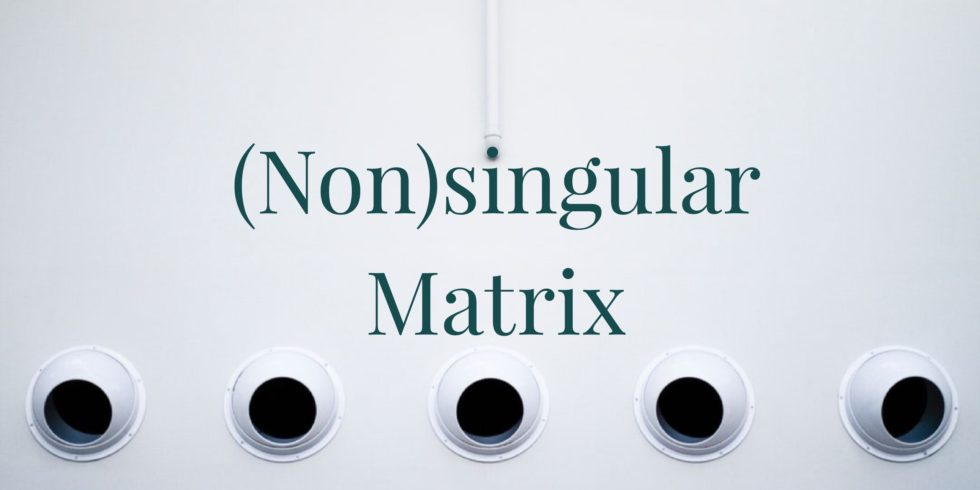# Prove that the Center of Matrices is a Subspace## Problem 658

Let $V$ be the vector space of $n \times n$ matrices with real coefficients, and define
$W = \{ \mathbf{v} \in V \mid \mathbf{v} \mathbf{w} = \mathbf{w} \mathbf{v} \mbox{ for all } \mathbf{w} \in V \}.$ The set $W$ is called the center of $V$.

Prove that $W$ is a subspace of $V$.Add to solve later

## Proof.

We must show that $W$ satisfies the three criteria for vector subspaces.
Namely, the zero vector of $V$ is in $W$, and $W$ is closed under addition and scalar multiplication.

First, the zero element in $V$ is the matrix $\mathbf{0}$ whose entries are all $0$. For any other matrix $\mathbf{x} \in V$, we have $\mathbf{0} \mathbf{x} = \mathbf{0} = \mathbf{x} \mathbf{0}$. So we see that $\mathbf{0} \in W$.

Now suppose $\mathbf{v}, \mathbf{w} \in W$ and $c \in \mathbb{R}$. Then for any $\mathbf{x} \in V$, we have
$(\mathbf{v} + \mathbf{w} ) \mathbf{x} = \mathbf{v} \mathbf{x} + \mathbf{w} \mathbf{x} = \mathbf{x} \mathbf{v} + \mathbf{x} \mathbf{w} = \mathbf{x} ( \mathbf{v} + \mathbf{w} ),$ where the second equality follows because $\mathbf{v}$ and $\mathbf{w}$ lie in $W$. So we see that $\mathbf{v} + \mathbf{w} \in W$ as well, and so $W$ is closed under addition.

Finally we must show that $c \mathbf{v} \in W$ as well. For any other $\mathbf{x} \in V$, we have
$(c \mathbf{v} ) \mathbf{x} = c ( \mathbf{v} \mathbf{x}) = c ( \mathbf{x} \mathbf{v} ) = \mathbf{x} ( c \mathbf{v} ),$ where the second equality follows from the fact that $\mathbf{v} \in W$ and so $\mathbf{v} \mathbf{x} = \mathbf{x} \mathbf{v}$.

Thus we see that $c \mathbf{v} \in W$, finishing the proof.Add to solve later

### More from my site

#### You may also like...

This site uses Akismet to reduce spam. Learn how your comment data is processed.

###### More in Linear Algebra##### If $M, P$ are Nonsingular, then Exists a Matrix $N$ such that $MN=P$

Suppose that $M, P$ are two $n \times n$ non-singular matrix. Prove that there is a matrix $N$ such that...

Close